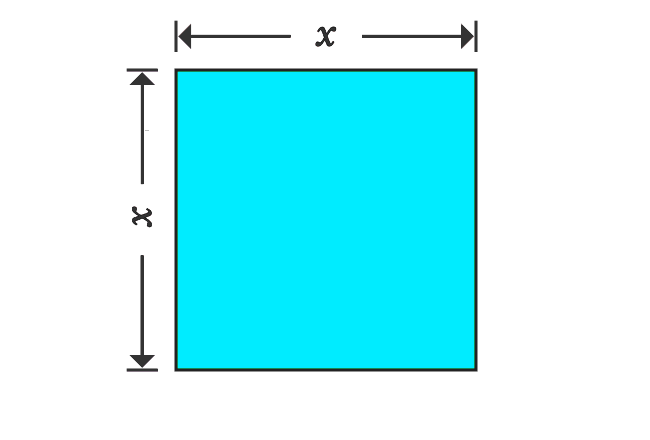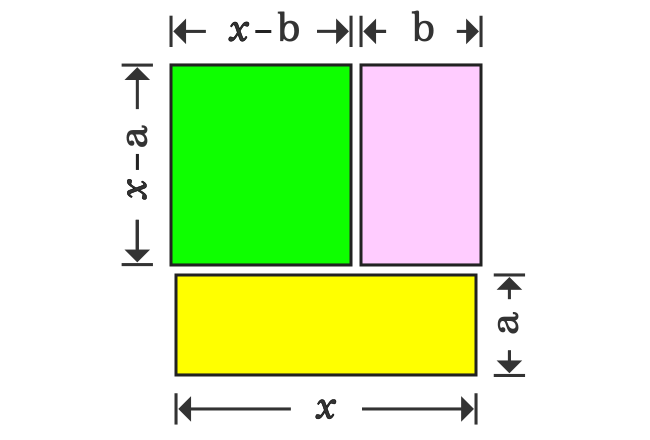# Proof of $(x-a)(x-b)$ identity in Geometric Method

The (x-a)(x-b) formula can be derived in geometrical approach by splitting a square as three different rectangles. Let’s start deriving the expansion of special product of binomials $x-a$ and $x-b$ in algebraic form.

### Express Area of SquareTake, a square and it is taken that the length of each side is $x$. Now, evaluate the area of a square mathematically.

$Area$ $\,=\,$ $x \times x$

$\implies$ $Area$ $\,=\,$ $x^2$

Therefore, the area of this square is equal to $x^2$ mathematically and remember this.

### Express Areas of Rectangles1. Now, divide the square horizontally as two different rectangles. If the width of one rectangle is $a$, then the width of the remaining rectangle is equal to $x-a$.
2. Similarly, divide the upper rectangle vertically as two different rectangles. If length of one rectangle is $b$, then the length of remaining rectangle is $x-b$.

Thus, a square whose area is $x^2$, is split as three different rectangles. Now, write the area of each rectangle in mathematical form.1. The length and width of first rectangle are $x-b$ and $x-a$ respectively, then its area is $(x-b)(x-a)$ in product form.
2. The length and width of second rectangle are $b$ and $x-a$ respectively, then its area in product form is $b(x-a)$.
3. The length and width of third rectangle are $a$ and $x$ respectively. Therefore, the area of this rectangle is equal to $ax$.

This information is used to derive the expansion for the special product of polynomials $(x-a)(x-b)$ geometrically.

### Proving the Algebraic identity

The square whose area is $x^2$, is divided as three different rectangles geometrically. Therefore, the area of a square is equal to sum of the areas of the rectangles.

$x^2$ $\,=\,$ $(x-b)(x-a)$ $+$ $b(x-a)$ $+$ $ax$

$\implies$ $x^2$ $\,=\,$ $(x-a)(x-b)$ $+$ $b(x-a)$ $+$ $ax$

$\implies$ $x^2$ $\,=\,$ $(x-a)(x-b)$ $+$ $bx-ba$ $+$ $ax$

$\implies$ $x^2$ $\,=\,$ $(x-a)(x-b)$ $+$ $ax+bx$ $-$ $ba$

$\implies$ $x^2$ $\,=\,$ $(x-a)(x-b)$ $+$ $ax+bx$ $-$ $ab$

$\implies$ $x^2$ $\,=\,$ $(x-a)(x-b)$ $+$ $(a+b)x$ $-$ $ab$

$\implies$ $x^2$ $-$ $(a+b)x$ $+$ $ab$ $\,=\,$ $(x-a)(x-b)$

$\,\,\, \therefore \,\,\,\,\,\,$ $(x-a)(x-b)$ $\,=\,$ $x^2$ $-$ $(a+b)x$ $+$ $ab$

Therefore, it is proved that the product of special binomials $x-a$ and $x-b$ is equal to the algebraic expression $x^2-(a+b)x+ab$.

This algebraic identity can also be derived directly in alternative method in geometry. The rectangle whose area is $(x-b)(x-a)$ can be obtained by subtracting the rectangles whose areas are $b(x-a)$ and $ax$ from the square whose area is $x^2$.

$(x-a)(x-b)$ $\,=\,$ $x^2$ $-$ $\Big(b(x-a)+ax\Big)$

$\implies$ $(x-a)(x-b)$ $\,=\,$ $x^2$ $-$ $\Big(bx-ba+ax\Big)$

$\implies$ $(x-a)(x-b)$ $\,=\,$ $x^2$ $-$ $\Big(ax+bx-ab\Big)$

$\implies$ $(x-a)(x-b)$ $\,=\,$ $x^2$ $-$ $\Big((a+b)x-ab\Big)$

$\,\,\, \therefore \,\,\,\,\,\,$ $(x-a)(x-b)$ $\,=\,$ $x^2-(a+b)x+ab$

Latest Math Topics
Jun 26, 2023
Jun 23, 2023

###### Math Questions

The math problems with solutions to learn how to solve a problem.

Learn solutions

Practice now

###### Math Videos

The math videos tutorials with visual graphics to learn every concept.

Watch now

###### Subscribe us

Get the latest math updates from the Math Doubts by subscribing us.